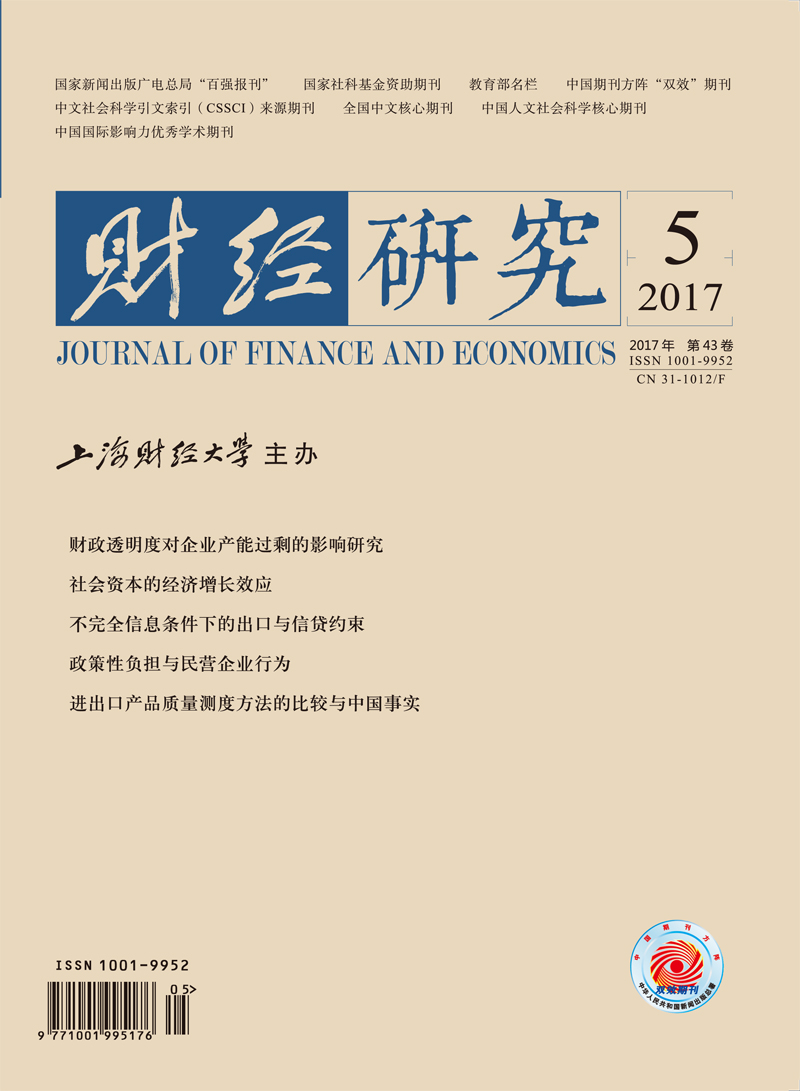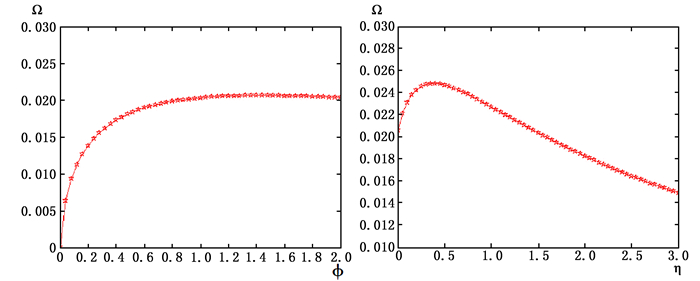﻿ 社会资本的经济增长效应
 财经研究2017, Vol. 43Issue (5): 31-430

#### 文章信息财经研究2017年43卷第5期

Zhang Liangliang, Yang Jun, Zhang Hua.

The impact of social capital on economic growth

Journal of Finance and Economics, 2017, 43(5): 31-43.

### 文章历史《财经研究》
2017第43卷第5期

The Impact of Social Capital on Economic Growth
Zhang Liangliang, Yang Jun, Zhang Hua
School of Economics and Business Administration, Chongqing University, Chongqing 400030, China
Abstract: Comprehensively and accurately exploring the impact of social capital on the long-term economic growth is of great practical significance to the enhancement of the status of social capital in the regional economic development. However, traditional endogenous growth model ignores the effect of time allocation on social capital accumulation and residents' utility. This paper introduces social capital into horizontal innovation growth model by using endogenous accumulation of social capital, and comprehensively considers the non-monotonicity impacts of social capital and leisure time on economic growth. Numerical simulations show that social capital plays a promotion role with a diminishing marginal effect in economic growth, but there is an inverse U-shape relationship between leisure time and economic growth, namely the appropriate increase in leisure time is beneficial to the accumulation of social capital. In order to verify the attribute of production factor of social capital, this paper constructs the measurement indexes of social capital at prefecture-level city level based on the network embeddedness feature of structural social capital. The empirical results show as follows: firstly, social capital has a significantly positive impact on economic growth, and its promotion role strengthens as urban economic development within regions increases; secondly, the output efficiency of social capital in the central and western regions is higher than the one in the eastern region, indirectly reflecting the current situation of excessive accumulation of social capital in the eastern region; thirdly, investment level and technological progress are two important ways to affect economic growth by social capital. These conclusions above provide useful reference for clarifying the economic attribute of social capital, stimulating the economic benefits of social capital, promoting the coordinated development of urban clusters and reducing regional differences.
Key words: social capital    economic growth    influence channel    urban agglomeration

(一) 最终产品部门

 $Y = L_Y^\alpha \int_0^A {x{{\left( i \right)}^{1-\alpha }}di}$ (1)

(二) 中间产品部门

(三) 研发部门

 $\dot A = \delta {L_A}{A^\beta }{S^{1-\beta }}$ (2)

(四) 家庭

 $U = \int_0^{ + \infty } {\frac{{{{\left( {c{S^\phi }L_s^\eta } \right)}^{1-\sigma }}-1}}{{1-\sigma }}{e^{ - \rho t}}dt}$ (3)

 $\dot K = rK + w\left( {1-{L_s}} \right)-{P_A}\dot A + \int_0^A {\pi di-c - I}$ (4)

Hemingway (1999) 在评述闲暇对实现民主政治的作用时，较早地提出“社会资本是闲暇效应的副产品”这一论点，并系统地回答了闲暇在社会资本形成、使用和维护中的角色地位问题。他认为闲暇过程中无意识的社会交往特征是社会网络持续存在和稳定延伸的基础，是信任、互惠性规范、公民行为准则等后续社会资本活动的先决条件。后继学者Antoci等 (2014) 遵循Hemingway (1999) 的开创性思路，设定社会资本运动方程为全社会平均闲暇时间的线性函数，却忽略了社会资本积累中家庭支出的作用及其对技术进步的溢出效应。本文的社会资本积累方程不仅考虑了家庭对社会资本的物质支出，还加入了社会资本基础存量和闲暇时间。社会资本运动方程为：

 $\dot S = \varepsilon {I^\theta }{S^{1-\theta }}{L_s}$ (5)

 $Y = L_Y^\alpha A{x^{1-\alpha }} = {\left( {A{L_Y}} \right)^\alpha }{K^{1-\alpha }}$ (6)
 $w = \frac{{\alpha Y}}{{{L_Y}}}, p = \frac{{\left( {1-\alpha } \right)Y}}{K}, \pi = \frac{{\alpha \left( {1-\alpha } \right)Y}}{A}$ (7)

 $\dot K = Y-c-I$ (8)
 $r = {\left( {1-\alpha } \right)^2}\frac{Y}{K} = {\left( {1-\alpha } \right)^2}{\left( {\frac{{A{L_Y}}}{K}} \right)^\alpha }$ (9)

 $H = \frac{{{{\left( {c{S^\phi }L_s^\eta } \right)}^{1- \sigma }}- 1}}{{1- \sigma }} + {\lambda _1}\left[{rK + w\left( {1-{L_s}} \right)-{P_A}\dot A + \int_0^A {\pi di-c - I} } \right] + {\lambda _2}\varepsilon {I^\theta }{S^{1 -\theta }}{L_s}$

 ${\lambda _1} = {c^{-\sigma }}{\left( {{S^\phi }L_s^\eta } \right)^{1-\sigma }}$ (10)
 ${\lambda _1} = {\lambda _2}\varepsilon \theta {L_s}{\left( {\frac{S}{I}} \right)^{1-\theta }}$ (11)
 $\eta c = w{L_s}-{\theta ^{-1}}I$ (12)
 $\frac{{{{\dot \lambda }_1}}}{{{\lambda _1}}} = \rho-r =-\sigma \frac{{\dot c}}{c} + \phi \left( {1-\sigma } \right)\frac{{\dot S}}{S}$ (13)
 $\frac{{\phi c}}{S} + \frac{{1- \theta }}{\theta }\frac{I}{S} = \frac{1}{{\varepsilon \theta {L_s}}}{\left( {\frac{I}{S}} \right)^{1- \theta }}\left[{\rho-\left( {1-\theta } \right)\frac{{\dot I}}{I}-\left( {\theta - 1 + \phi - \phi \sigma } \right)\frac{{\dot S}}{S} + \sigma \frac{{\dot c}}{c}} \right]$ (14)

 $\frac{{\phi c}}{S} + \frac{{1- \theta }}{\theta }\frac{I}{S} = \frac{1}{{\varepsilon \theta {L_s}}}{\left( {\frac{I}{S}} \right)^{1- \theta }}\left[{\rho-\left( {\phi-\phi \sigma-\sigma } \right)\mathit{\Omega }} \right]$ (15)

 $\frac{{{L_A}}}{{{L_Y}}} = \frac{{\left( {1-\alpha } \right)\mathit{\Omega }}}{r} = \frac{{\left( {1-\alpha } \right)\mathit{\Omega }}}{{\rho-\left( {\phi - \phi \sigma - \sigma } \right)\mathit{\Omega }}}$ (16)

 $\frac{K}{A} = {\left[{\frac{{{{\left( {1-\alpha } \right)}^2}}}{{\rho-\left( {\phi-\phi \sigma - \sigma } \right)\mathit{\Omega }}}} \right]^{\frac{1}{\alpha }}} \times {L_Y}$ (17)

 $\mathit{\Omega } = \frac{{\dot K}}{K} = {\left( {\frac{{A{L_Y}}}{K}} \right)^\alpha }-\frac{c}{K}-\frac{I}{K} = {\left( {\frac{{A{L_Y}}}{K}} \right)^\alpha }-\left( {\frac{c}{S} + \frac{I}{S}} \right)\frac{S}{K}$ (18)

 $\left( {\eta-\frac{{\alpha {L_s}}}{{{L_Y}}}} \right)\frac{c}{S}\frac{S}{K} = \frac{{\alpha {L_s}}}{{{L_Y}}}\mathit{\Omega } + \left( {\frac{{\alpha {L_s}}}{{{L_Y}}}-\frac{1}{\theta }} \right)\frac{I}{S}\frac{S}{K}$ (19)

 ${L_Y} + {L_A} + {L_S} = 1$ (20)图 1 社会资本偏好、闲暇时间偏好对经济增长的影响

(一) 社会资本指标

(二) 其他变量指标

(三) 数据来源与说明

(一) 全样本空间面板模型

① 城市间距通过国家基础地理信息系统网站 (http://www.ngcc.cn/) 提供的电子地图测度得到。

① 限于篇幅，没有报告Moran’I的计算结果，备索。

 行政邻接矩阵 距离权重矩阵 (1) (2) (3) (4) (5) (6) (7) (8) Telephone 0.0259*** 0.0214*** 0.0237*** 0.0260*** (0.01) (0.01) (0.01) (0.01) Internet 0.7150*** 0.6397*** 0.5225*** 0.6781*** (0.01) (0.01) (0.01) (0.01) Capital 0.1748*** 0.1626*** 0.1607*** 0.1510*** 0.1497*** 0.1563*** 0.1416*** 0.1469*** (0.00) (0.00) (0.00) (0.00) (0.00) (0.00) (0.00) (0.00) Technology 0.0509 0.0024 0.0895* 0.0162 0.1216*** 0.1506*** 0.1498*** 0.1655*** (0.05) (0.05) (0.05) (0.05) (0.05) (0.05) (0.05) (0.05) Industry -2.6523*** -3.1260*** -2.6753*** -3.1232*** -2.5753*** -3.0286*** -2.6001*** -3.0248*** (0.08) (0.08) (0.08) (0.07) (0.07) (0.08) (0.07) (0.08) Infrastructure 0.0097 0.1207*** 0.0023 0.1080*** -0.0270** 0.0597*** -0.0291** 0.0492*** (0.01) (0.01) (0.01) (0.01) (0.01) (0.02) (0.01) (0.02) Finance -0.1570*** -0.0949*** -0.1630*** -0.1024*** -0.1628*** -0.1575*** -0.1659*** -0.1620*** (0.02) (0.02) (0.02) (0.02) (0.02) (0.02) (0.02) (0.02) Open 0.3190*** 0.3194*** 0.2429*** 0.2504*** 0.2198*** 0.2626*** 0.1693*** 0.2154*** (0.01) (0.01) (0.02) (0.02) (0.01) (0.02) (0.02) (0.0181) Government -1.1470*** -1.2513*** -1.1603*** -1.2639*** -1.1376*** -1.1556*** -1.1444*** -1.1585*** (0.06) (0.07) (0.06) (0.07) (0.06) (0.08) (0.06) (0.08) City 0.3188*** 0.2069*** 0.2632*** 0.1603*** 0.3829*** 0.2965*** 0.3381*** 0.2382*** (0.02) (0.02) (0.02) (0.02) (0.02) (0.02) (0.02) (0.02) Rho 0.3915*** 0.3840*** 0.5229*** 0.5094*** (0.01) (0.01) (0.01) (0.01) Lambda 0.7150*** 0.7122*** 0.7796*** 0.7667*** (0.01) (0.01) (0.02) (0.02) _cons 6.1154*** 9.9656*** 6.1690*** 9.9627*** 4.8663*** 10.1227*** 4.9797*** 10.0975*** (0.11) (0.06) (0.11) (0.06) (0.13) (0.07) (0.12) (0.06) LogL -529.4511 -363.0696 -470.1184 -312.6695 -369.0311 -388.5673 -338.1146 -342.6834 注：***、**和*分别表示在1%、5%和10%的水平上显著；括号中的系数表示标准差。下表同。

(二) 分区域面板分位数模型

 均值回归 q10 q25 q50 q75 q90 全国 Telephone 0.0903***(0.01) 0.0579*(0.03) 0.0597**(0.03) 0.0756*(0.05) 0.1313**(0.05) 0.1242**(0.05) Internet 0.5367***(0.04) 0.6248**(0.29) 0.7302**(0.34) 1.1802***(0.46) 1.9873***(0.52) 2.4854***(0.67) 东部 Telephone 0.0687**(0.03) 0.0378*(0.02) 0.0139**(0.01) 0.0126*(0.01) 0.0101*(0.01) 0.0072(0.04) Internet 0.6135***(0.05) 0.3591**(0.15) 0.3001*(0.19) 0.5453**(0.26) 0.7252**(0.36) 0.7240**(0.36) 中部 Telephone 0.0237*(0.01) 0.0177(0.08) 0.0368(0.11) 0.0967**(0.05) 0.1161**(0.06) 0.0657**(0.03) Internet 2.9932***(0.14) 2.8377***(0.80) 3.0974***(0.82) 3.4740***(0.98) 4.3482***(1.50) 6.1549***(1.96) 西部 Telephone 0.1758***(0.02) 0.1681**(0.09) 0.2829**(0.15) 0.3880***(0.13) 0.4008***(0.10) 0.5303***(0.14) Internet 1.7096***(0.10) 1.0470**(0.46) 1.3828**(0.78) 3.0713***(1.19) 3.8388***(1.21) 3.9845**(1.49) 注：表中结果通过R软件中的rqpd_0.6程序包得出。限于篇幅，这里只给出了核心解释变量的相关结果。

① 这里的潜台词是：以互联网使用情况衡量的社会资本Ⅱ在形式上比以电话用户衡量的社会资本Ⅰ更为高级和紧跟时代，即互联网技术的普及给社会资本影响经济增长的相关机制带来了颠覆性的结构变化 (Salahuddin等，2016)。当然，这一论断还需要中国的现实数据去验证。

(三) 社会资本影响经济增长的渠道

  程宇丹, 龚六堂. 外债的经济增长效应与影响渠道——发达国家与发展中国家比较[J]. 数量经济技术经济研究, 2015(10): 108–123.  高正平, 张兴巍. 社会资本、政府治理与区域企业自生能力——基于我国省际数据的实证研究[J]. 财贸经济, 2013(9): 121–129.  林洲钰, 林汉川. 中国制造业企业的技术创新活动——社会资本的作用[J]. 数量经济技术经济研究, 2012(10): 37–51.  刘灿, 金丹. 社会资本与区域经济增长关系研究评述[J]. 经济学动态, 2011(6): 73–77.  魏翔, 虞义华. 闲暇效应对经济产出和技术效率的影响[J]. 中国工业经济, 2011(1): 130–139.  严成樑. 社会资本、创新与长期经济增长[J]. 经济研究, 2012(11): 48–60. DOI:10.3969/j.issn.1004-7778.2012.11.016  周亚虹, 宗庆庆, 陈曦明. 财政分权体制下地级市政府教育支出的标尺竞争[J]. 经济研究, 2013(11): 127–139. DOI:10.3969/j.issn.1002-5863.2013.11.059  Akcomak I S, Weel B. Social capital, innovation and growth:Evidence from Europe[J]. European Economic Review, 2009, 53(5): 544–567. DOI:10.1016/j.euroecorev.2008.10.001  Anchorena J, Anjos F. Social ties and economic development[J]. Journal of Macroeconomics, 2015, 45: 63–84. DOI:10.1016/j.jmacro.2015.04.004  Antoci A, Guerrini L, Sodini M, et al. A two-sector model of economic growth with social capital accumulation[J]. Journal of Behavioral and Experimental Economics, 2014, 53: 56–65. DOI:10.1016/j.socec.2014.08.002  Carmona-Lavado A, Cuevas-Rodriguez G, Cabello-Medina C. Social and organizational capital: Building the context for innovation[J]. Industrial Marketing Management, 2010, 39(4): 681–690. DOI:10.1016/j.indmarman.2009.09.003  Chou Y K. Three simple models of social capital and economic growth[J]. The Journal of Socio-Economics, 2006, 35(5): 889–912. DOI:10.1016/j.socec.2005.11.053  Hemingway J L. Leisure, social capital, and democratic citizenship[J]. Journal of Leisure Research, 1999, 31(2): 150–165.  Lucas R E. On the mechanics of economic development[J]. Journal of Monetary Economics, 1988, 22(1): 3–42. DOI:10.1016/0304-3932(88)90168-7  Romer P M. Endogenous technological change[J]. Journal of Political Economy, 1990, 98(5): 71–102. DOI:10.1086/261725  Salahuddin M, Tisdell C, Burton L, et al. Does internet stimulate the accumulation of social capital? A macro-perspective from Australia[J]. Economic Analysis and Policy, 2016, 49: 43–55. DOI:10.1016/j.eap.2015.11.011